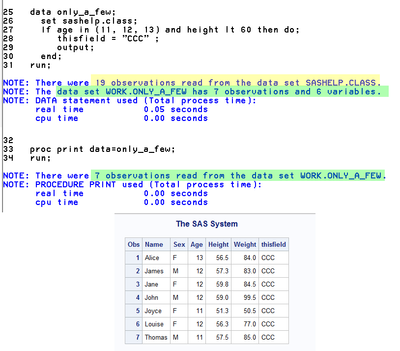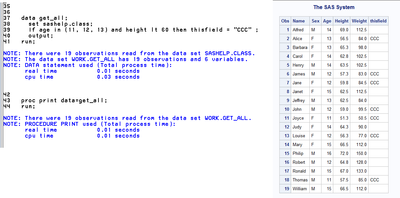## Conditionally output observations only

Hello team,

I want to conditionally output observations not data (This important to pay attention, it is observations not datasets). How should I go for it?

```data want;
set have;
If member in ("A", "B) and code = "T" and payer in ("V") then thisfield = "CCC" ;
run;

or
data want;
set have;
If member in ("A", "B) and code = "T" and payer in ("V") then do;
thisfield = "CCC" ;
output; /* I need the observations written to dataset */
run;```

This question differs from my previous post. I am not splitting data but conditionally output observations to one dataset.

Can I use output statements to write observations to one single dataset?

Thanks,

blue & blue

Blue Blue
4 REPLIES 4

## Re: Conditionally output observations only

Hi:

Consider this code.SASHELP.CLASS has 19 rows with ages spanning from 11 to 16. In this code, I am outputting only the rows that meet the condition. However, my output is INSIDE the IF/THEN DO/END block because I want to do 2 things when the condition is met: 1) create the variable THISFIELD when the condition is true and 2) output the entire observation to the output data set when the condition is true.

Compare that code to this code where the output is being used for every row:In this example, you can see that all 19 observations were written to the output dataset in this case. However, only the rows that met the IF condition have a value for the THISFIELD variable. The rest of the rows have a blank for the THISFIELD variable.

The difference is that the first program is executing 2 statements when the condition is met. The second program is only executing the assignment statement that created THISFIELD when the condition is met. For the second program, the OUTPUT statement is operating on EVERY row.

Both types of output are under your control based on how you code your conditional logic. A WHERE statement would also work for my example:A WHERE works for my example because the variables used in the WHERE already exist in the input data set. So in this case, the only observations that are passed to the program are the observations that meet the WHERE condition. So I know that these rows are the ones that should be output and should have THISFIELD created.

Cynthia

## Re: Conditionally output observations only

It is good to know the difference. I need to test it.
I am thankful to you!
Blue
Blue Blue

## Re: Conditionally output observations only

There is no condition on your output statement in this code:

```data want;
set have;
If member in ("A", "B) and code = "T" and payer in ("V") then do;
thisfield = "CCC" ;
output; /* I need the observations written to dataset */
run;```

In fact it should generate an ERROR of an unclosed DO block.

Which is one reason that we often ask for LOG entries of submitted code.

If you expect the above to only write to the output data set when the conditions member in ("A", "B) and code = "T" and payer in ("V") are true, then one way the code would look is:

```data want;
set have;
If member in ("A", "B) and code = "T" and payer in ("V") then do;
thisfield = "CCC" ;
output; /* I need the observations written to dataset */
end; /* this closes the DO block*/
run;```

You can use Output as the result of any comparison

`If thisvar=2 and thatvar='ABC' then output;`

IF you use one output and have multiple other conditions you have to provide the explicit output statement for each condition as the implied Output statement that normally executes at the end of a data step for an observation does not execute.

You really want to carefully examine examine your requirements as if the conditions overlap you will get the same observation more than once.

```data want;
set have;
If member in ("A", "B) and code = "T" and payer in ("V") then do;
thisfield = "CCC" ;
output; /* I need the observations written to dataset */
end;
/* could duplicate the above observation when the Member is 'A' */
if member in ("A", "C") then output;
/* Would also create duplicates for the first*/
if  code = "T" and payer in ("V") then do;
otherfield='QQQ';
output;
end;
run;```

## Re: Conditionally output observations only

You could let SAS filter the observations on the way INTO the data step.

``````data want;
set have;
where  member in ("A", "B) and code = "T" and payer in ("V");
thisfield = "CCC" ;
run;``````

You could use a subsetting IF statement. (required when the condition is based on something calculated in this step).

``````data want;
set have;
if member in ("A", "B) and code = "T" and payer in ("V");
thisfield = "CCC" ;
run;``````

Or use the opposite condition to conditionally DELETE the observation.

``````data want;
set have;
if not (member in ("A", "B) and code = "T" and payer in ("V")) then delete;
thisfield = "CCC" ;
run;``````

You could conditionally write the observation in the MIDDLE of the data step (this is your second example with the missing END statement added).

``````data want;
set have;
if member in ("A", "B) and code = "T" and payer in ("V") then do;
thisfield = "CCC" ;
output;
end;
run;``````

Which could more easily be done like this instead:

``````data want;
set have;
thisfield = "CCC" ;
if member in ("A", "B) and code = "T" and payer in ("V") then output;
run;``````
Discussion stats
• 4 replies
• 354 views
• 3 likes
• 4 in conversation